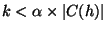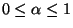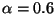Next: Merging Groups Up: Role Classification Previous: Role Classification

## Forming Groups

Group formation can be thought of as a graph theory problem. From the connection sets of I, one can generate a neighborhood graph, nbh-graph, where each node represents a host and each edge with weight e represents that there are e common neighbors between the hosts. Thus, a neighborhood graph captures the extent to which pairs of hosts communicate with the same set of other hosts. We use an undirected graph since almost all communication between hosts in the intranets is bi-directional. However, in certain situations, directionality may be used to improve the quality of the grouping results and we continue to investigate this issue. One approach to the grouping problem is to treat it as a k-clique problem where nbh-graph is partitioned into cliques of size k in which each edge in the clique has a weight greater than or equal to some constant c. Once a k-clique is identified, one assigns all the nodes in the k-clique to one group, since they all share at least c common neighbors. This approach is problematic, because (i) the k-clique problem is NP-complete , and (ii) requiring that each host pair in a group has exactly k common neighbors is too strong a requirement. Another approach is to treat grouping as related to the problem of identifying bi-connected components (BCCs). A BCC is a connected component in which any two edges lie in a simple cycle. Thus, there exist at least two disjoint paths between any two nodes in a BCC. Unlike the k-clique problem, BCC can be solved in O(N+E), where N and E are the number of nodes and edges in the graph respectively [9,27]. Moreover, all nodes in the BCC need not be connected to each other directly. This approach is the one we use. The group formation phase operates as follows:
1.
Generate the connectivity graph, conn-graph, based on the observed connection patterns.
2.
For k=kmax down to 1, where kmax is the maximum number of hosts with which a single host communicates:
Repeat until no new groups can be assigned:
1. From conn-graph, build the k-neighborhood graph k-nbh-graph.
2. Remove group nodes (see 2d) from k-nbh-graph.
3. Generate all BCCs in k-nbh-graph.
4. For each BCC B, replace in conn-graph the nodes in B by a new group node G representing those nodes. Label G by a pair (IDG, KG), where IDG is a unique identifier and KG is k. (KG will be used later to compute the degree of similarity between groups.)
5. For each ungrouped host h, whereand, create a new group G containing only h as described in 2d.
The algorithm runs iteratively over conn-graph until no ungrouped node remains or k = 0. At each step multiple BCCs may be identified simultaneously and a single node could be a part of several BCCs indicating that it may share multiple roles. In this case, the node becomes a part of a BCC with the largest size. If more than one such BCC exists, we choose one randomly. By iterating over k from high to low, the algorithm associates each host h with other hosts with the strongest similarity. In the grouping algorithm, the minimum number of nodes required to form a BCC is two. Technically, the minimum number of nodes to form a BCC is 3, since we do not allow duplicate edges between any two nodes. Nevertheless, we allow two isolated nodes connected by an edge to form a group. Since a BCC is not a clique, some node pairs in the BCC may not have edges between them allowing node pairs that do not share at least k common neighbors to be in the same group. However, any two nodes in a BCC have at least two disjoint paths along which two successive nodes share at least k common neighbors. In other words, any two nodes in a group demonstrate in at least two different ways that they have strong similarity in connection habits, significantly reducing the possibility that they may serve different roles. This observation is a major reason why we believe BCCs are suitable for forming groups. When a set of hosts is placed into a group, the nodes representing those hosts are removed from conn-graph and replaced by one node (called the group node) representing the entire group. There are edges connecting that group node to each node to which one of the hosts in the group was connected. In some cases where a node may have connection patterns so different from any other nodes, the node should form a group by itself. Step 2e forms a new group with only h in it if there exist no nodes that have the number of common neighbors greater than or equal to. We setand find that it works well with various networks.Figure 2 illustrates the evolution of the grouping algorithm, in terms of k-nbh-graph, for the network depicted in Figure 1. The first group is formed when k = M + N, where M is the number of hosts used by sales personnels and N is the number of hosts used by engineers. For specificity, let us assume that M = N = 3. As shown in the picture, the 6-nbh-graph contains two hosts, Mail and Web, and the algorithm puts them in one group. When k = 3, the algorithm identifies two additional BCCs, one containing all the sales machines and the other, all the engineering machines. Finally, because of the bootstrap condition (see Step 2e), the algorithm creates two groups, one containing SalesDatabase and the other, SourceRevisionControl, when k = 1 < 0.6 x M.Next: Merging Groups Up: Role Classification Previous: Role Classification
Godfrey Tan 2003-04-01